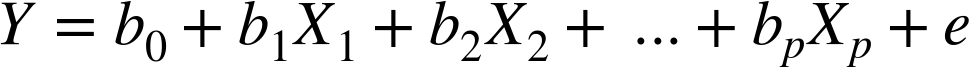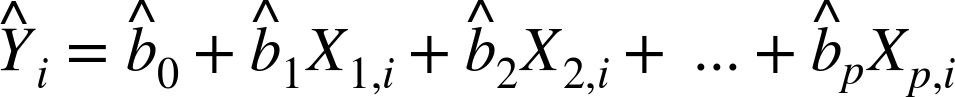# Data Science Algorithms: Multiple Linear Regression

04 Jul
04Jul

### Introduction

So, in my previous blog on simple linear regression we have seen that, when there are more than one independent (Exploratory) variables then its a Multiple linear regression.

So definitely, as more than one Independent variable are involved it is quite obvious to have multiple predictors and in turn instead of a line we will get linear model like that gives relationship between each coefficient and its variable (feature) is linear.

### Mathematical EquationAll of the other concepts in simple linear regression, such as fitting by least squares and the definition of fitted values and residuals, extend to the multiple linear regression.

For example, the fitted values are given by: